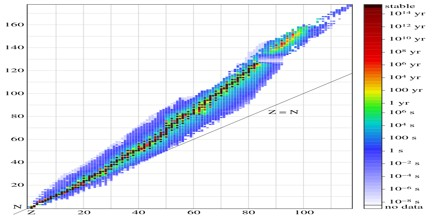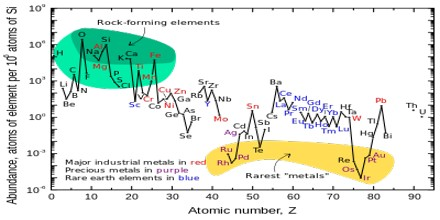Definition

Primordial Nuclides or primordial isotopes are nuclides found on the earth that have existed in their current form since before Earth was formed, according to stellar evolution theory. Due to the age of the Earth of 4.58×109 years, this means that the half-life of the given nuclides must be greater than about 5×107 years for practical considerations. E.g. for a nuclide with half-life 6×107 years, this means 77 half-lives have elapsed, meaning that for each mole (6.02×1023 nuclide) of that nuclide being present at the formation of earth, only 6 atoms remain today.Primordial Nuclides were present in the interstellar medium from which the solar system was formed, and were formed in the Big Bang, by nucleosynthesis in stars and supernovae followed by mass ejection, by cosmic ray spallation, and potentially from other processes. They are the stable nuclides plus the long-lived fraction of radionuclides surviving in the primordial solar nebula through planet accretion until the present. Only 286 such nuclides are known.

It is estimated that about 32 primordial nuclides are radioactive, it becomes very difficult to determine the exact total number of radioactive primordials, because the total number of stable nuclides is uncertain. There exist many extremely long-lived nuclides whose half-lives are still unknown.

Some unstable isotopes which occur naturally (such as 14C, 3H, and 239Pu) are not primordial, as they must be constantly regenerated. This occurs by cosmic radiation, in the case of cosmogenic nuclides such as 14C and 3H, or (rarely) by such processes as geonuclear transmutation (neutron capture of uranium in the case of 239Pu).

Elements of Primordial Nuclide

There are 254 stable primordial nuclides and 32 radioactive primordial nuclides, but only 80 primordial stable elements (1 through 82, i.e. hydrogen through lead, exclusive of 43 and 61, technetium and promethium respectively) and three radioactive primordial elements (bismuth, thorium, and uranium). Bismuth’s half-life is so long that it is often classed with the 80 primordial stable elements instead, since its radioactivity is not a cause for serious concern. The numbers of elements are smaller, because many primordial elements are represented by more than one primordial nuclide.In theory, spontaneous fission is possible for all elements with atomic numbers >40, but has not been observed for most elements up to lead. The list lists nuclides unstable only to this fission mechanism, before nuclides theoretically unstable to additional mechanisms. Those that have been checked for radioactivity are indicated with “> number” and show the lower limit for the half-life based on experimental observation. Such nuclides are considered to be “stable” until decay has been observed in some fashion. For example, tellurium-123 was reported to be radioactive, but the same experimental group later retracted this report, and it presently remains observationally stable.

Nuclide identifiers are given by their atomic mass number A and the symbol for the corresponding chemical element, corresponding to the unique proton number. In the cases that this is not the ground state, this is indicated by a m for metastable appended to the mass number.32 Primordial Nuclides represent radioisotopes of 28 distinct chemical elements (cadmium, neodymium, samarium, tellurium, and uranium each have two primordial radioisotopes). The radionuclides are listed in order of stability, with the longest half-life beginning the list. These radionuclides in many cases are so nearly stable that they compete for abundance with stable isotopes of their respective elements. For three chemical elements, a very long lived radioactive primordial nuclide is found to be the most abundant nuclide for an element that also has a stable nuclide. These unusual elements are tellurium, indium, and rhenium.

List Legends of Primordial Nuclide

Nuclide Column: Nuclide identifiers are given by their mass number A and the symbol for the corresponding chemical element (implies a unique proton number).

Energy Column: The column labeled “energy” denotes the mass of the average nucleon of this nuclide relative to the mass of a neutron (so all nuclides get a positive value) in MeV/c2, formally: mn − mnuclide / A.

Half-life Column: All times are given in years

Decay Mode Column

α          α decay

β−        β− decay

K         electron capture

KK      double electron capture

β+        β+ decay

SF        spontaneous fission

2 β−     double β− decay

β+β+    double β+ decay

I           isomeric transition

p          proton emission

n          neutron emission